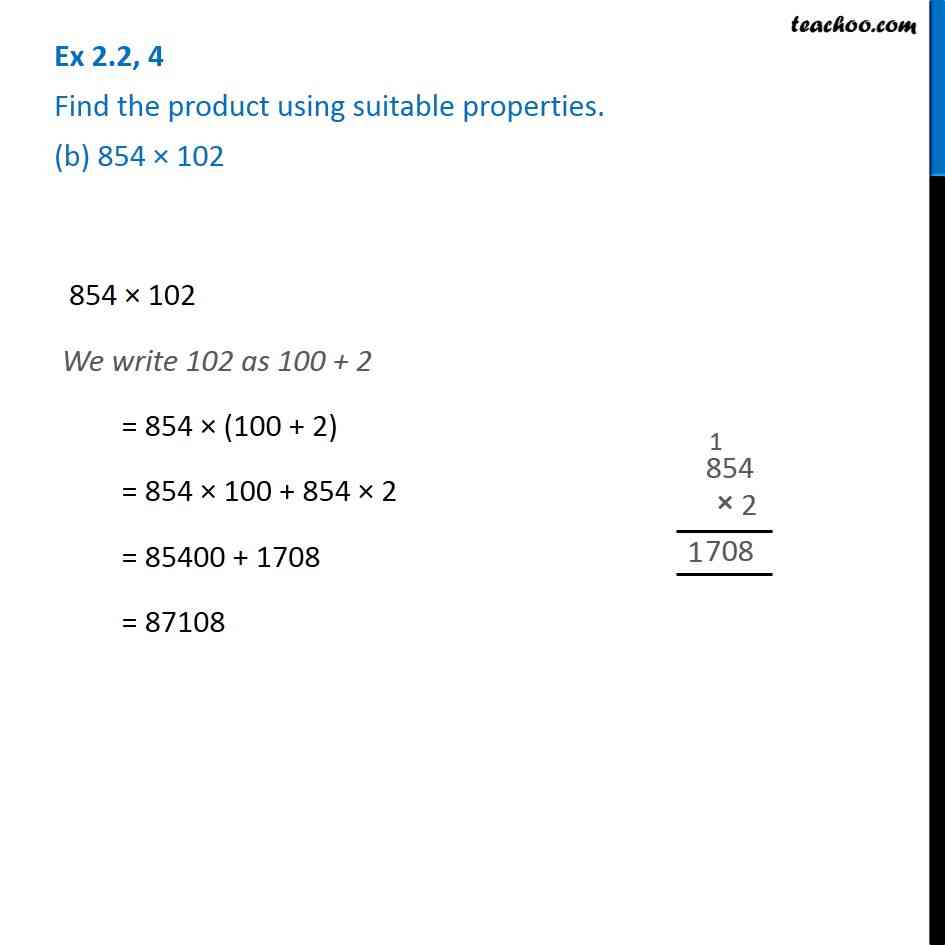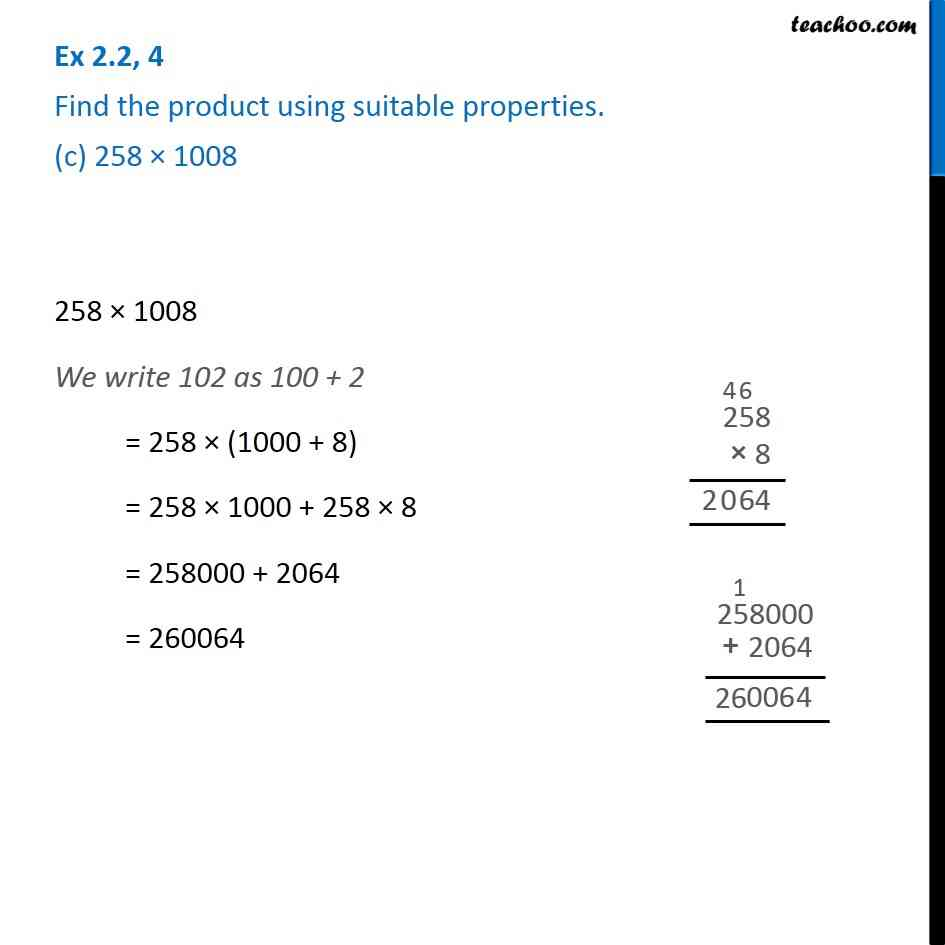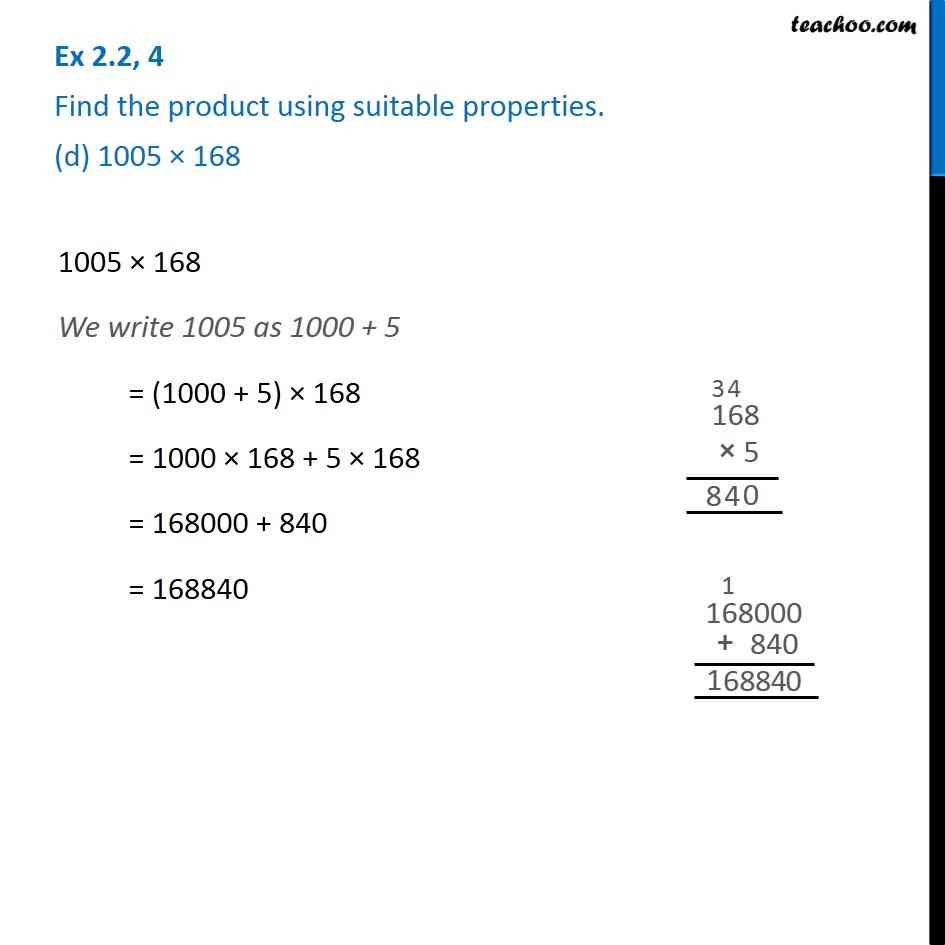Subscribe to our Youtube Channel - https://you.tube/teachoo

1. Chapter 2 Class 6 Whole Numbers
2. Concept wise
3. Distributivity

Transcript

Ex 2.2, 4 Find the product using suitable properties. (a) 738 × 103 738 × 103 We write 103 as (100 + 3) = 738 × (100 + 3) = 738 × 100 + 738 x 3 = 73800 + 2214 = 76014 Ex 2.2, 4 Find the product using suitable properties. (b) 854 × 102 854 × 102 We write 102 as 100 + 2 = 854 × (100 + 2) = 854 × 100 + 854 × 2 = 85400 + 1708 = 87108 Ex 2.2, 4 Find the product using suitable properties. (c) 258 × 1008 258 × 1008 We write 102 as 100 + 2 = 258 × (1000 + 8) = 258 × 1000 + 258 × 8 = 258000 + 2064 = 260064 Ex 2.2, 4 Find the product using suitable properties. (d) 1005 × 168 1005 × 168 We write 1005 as 1000 + 5 = (1000 + 5) × 168 = 1000 × 168 + 5 × 168 = 168000 + 840 = 168840

Distributivity